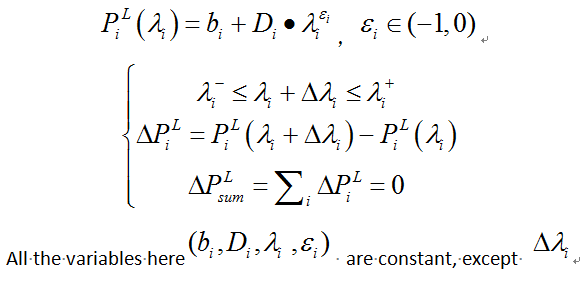# How can I solve this problem by cvx solver?

I want to solve a problem like this, I just want to get a feasible solution at first step.PiL is a concave function.

#####################
Here is my cvx code piece

``````cvx_begin
y0 = b + D .* pow_p(lamda,e);
y1 = b + D .* pow_p(lamda+x,e);

subject to
RTP_Price_min<= lamda+x <=RTP_Price_max
sum(y1 - y0) <= 1e-4
sum(y1 - y0) >= -1e-4
cvx_end
``````

#################
Here is error information.

Warning: A non-empty cvx problem already exists in this scope.
It is being overwritten.

In cvxprob.cvxprob at 28
In cvx_begin at 41
In test_cvx at 50
??? Error using ==> cvxprob.newcnstr at 192
Disciplined convex programming error:
Invalid constraint: {convex} >= {real constant}

Error in ==> cvx.ge at 21
b = newcnstr( evalin( ‘caller’, ‘cvx_problem’, ‘[]’ ), x, y, ‘>=’ );

Error in ==> test_cvx at 58
sum(y1 - y0) >= -1e-4

Any help would be appreciated.

thanks a lot, I just change the style of writing.
But how can I express some constrains like:
x>=1 or x<=-2
I couldn’t find the symbol to express “or” and I couldn’t use expressions like “abs(x)>= constant”

You can handle
`x>=1 or x<=-2`
by brute force, by solving separate problems for x>=1 and for x <= 2 (x is presumably a vector with dimension > 1, or else in this example, the “or” is always satisfied), and taking as your solution, the best solution of the separate problems. If you have several instances of “or”, this approach would result in a combinatorial blow up. I think you could alternatively use the mixed integer capability of CVX.

As for abs(x) >= constant, that is (very) non-convex (unless the constant is zero). So of course CVX won’t let you use that.

Read the FAQ again. CVX is the wrong tool for you.

Thanks a lot. The variable x is a scalar in simple situation, but might also be a vector with huge dimensions, so maybe I would choose another toolbox.